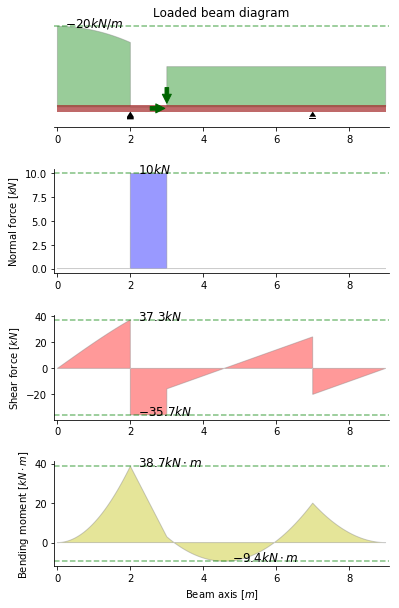Beam bending calculator¶

• Leave lines 1-6 and 21-23 as they are; they ensure that all necessary modules are imported and active.

• Whatever code is written to the right of the numeral sign (#) is a comment. These comments are not executed, so you can safely ignore or delete them.

The current code reproduces this example.

In :
# Initialization (leave this section as it is, unless you know what you are doing)
!pip install -q beambending
%matplotlib inline

# Go on and play around with the values in this section. See what happens!
### ------------------------------------------------------------------------ ###
beam = Beam(9)
beam.pinned_support = 2    # x-coordinate of the pinned support
beam.rolling_support = 7  # x-coordinate of the rolling support
PointLoadH(10, 3),  # 10kN pointing right, at x=3m
PointLoadV(-20, 3),  # 20kN downwards, at x=3m
DistributedLoadV(-10, (3, 9)),  # 10 kN/m, downwards, for 3m <= x <= 9m
DistributedLoadV(-20 + x**2, (0, 2)),  # variable load, for 0m <= x <= 2m
))
### ------------------------------------------------------------------------ ###

# Output generation (leave this section as it is, unless you know what you are doing)
fig = beam.plot()
fig.savefig("./results.pdf")By the way, a double asterisk is how you write a power in Python: "2 * x**2 + cos(5)" means $2x^2 + \cos(5)$